# Algebra 1 : How to divide monomial quotients

## Example Questions

### Example Question #11 : Simplifying Exponents

Simplify the rational expression.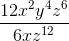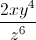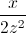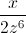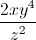Explanation:To simplify variables with exponents through division, you must subtract the exponent in the denominator from the numerator.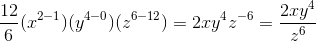Remember that negative exponents will eventually be moved back to the denominator.

### Example Question #78 : Expressions

Divide the fractions.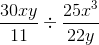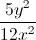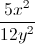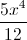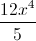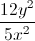Explanation:Dividing fractions is equal to multiplying by the reciprocal.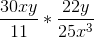Cross-cancel the 30 with the 25, and the 22 with the 11.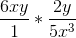Combine via multiplication and simplify.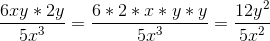### Example Question #14 : Simplifying Exponents

Simplify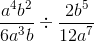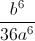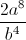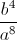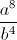Explanation:

Rewrite so that you are multiplying the reciprocal of the second fraction: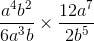You can then simplify using rules of exponents: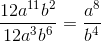### Example Question #51 : Monomials

Evaluate: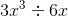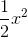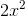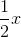Explanation:

First, divide the coefficients.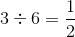.

Now consider the variables.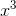means x times itself 3 times. Doing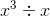is the same as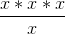.

We see that an x from the top can cancel with the x on the bottom, leaving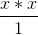, or. Our answer then is.

### Example Question #52 : Monomials

Divide: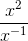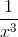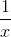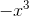Explanation:

Dividing exponents by the same base allows the powers to be subtracted.  Rewrite the exponents as one base.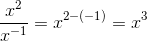### Example Question #53 : Monomials

Find the result of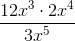.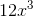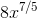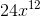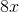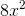Explanation:

The question asks for the quotient of.

We can rewrite the expression using the rules of multiplication and exponents to find the answer of.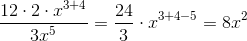### Example Question #61 : Monomials

Divide: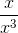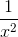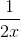Explanation:

Notice that both bases are the same.  The power of the base in the numerator is one.

Since the bases are divided, simply subtract the powers.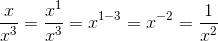### Example Question #62 : Monomials

Divide the following monomial quotients: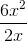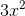Explanation:

To solve this problem, split it into two steps:

1. Divide the coefficients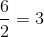2. Divide the variables. We also need to remember the following laws of exponents rule: When dividing variables, subtract the exponents.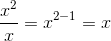Combine these to get the final answer:### Example Question #63 : Monomials

Divide the following monomial quotients: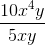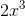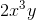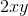Explanation:

To solve this problem, split it into two steps:

1. Divide the coefficients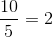2. Divide the variables. We also need to remember the following laws of exponents rule: When dividing variables, subtract the exponents.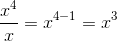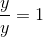Combine these to get the final answer:### Example Question #64 : Monomials

Divide the following monomial quotients: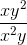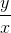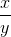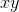Explanation:

To solve this problem, divide the variables. We also need to remember the following laws of exponents rule: When dividing variables, subtract the exponents.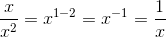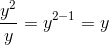Combine these to get the final answer: